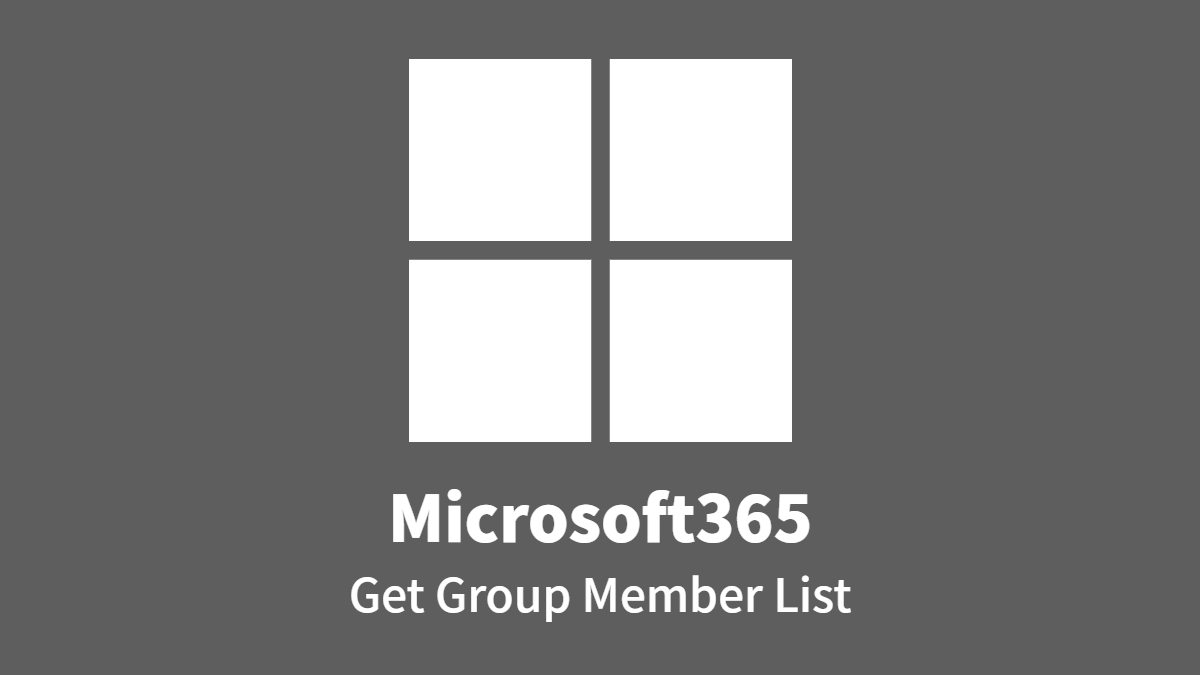Microsoft365: Get Group Member List
Retrieves a list of members of a Microsoft365 group. Up to 999 lists can be retrieved.
Configs
• A: OAuth2 Setting *
• B: target Group ID *#{EL}
• C: List Count (default:100, MAX:999)#{EL}
• D: Group Member List (update) *
• : next link URL (update)
• X: Access Log (update)
Script (click to open)
``````

// Get Microsoft365 Group Member List via Microsoft Graph API v1.0 (ver. 202211)
// (c) 2022, Questetra, Inc. (the MIT License)

//// == Config Retrieving ==
const oauth2  = configs.get( "conf_OAuth2" ) + "";

const groupId = configs.get( "conf_groupId" ) + "";
let count = 100;
if ( configs.get( "conf_count" ) ) {
count = configs.get( "conf_count" ) + "";
}

const dataId_list     = configs.get( "conf_list" );
const dataId_log      = configs.get( "conf_log" );

//// == Calculating ==
let accessLog = "";
let uri = "https://graph.microsoft.com/v1.0/groups/" + groupId + "/members?\$top=" + count;
let response = httpClient.begin()
.authSetting( oauth2 )
.get( uri );
accessLog += "---GET request--- " + response.getStatusCode() + "\n";
accessLog += response.getResponseAsString() + "\n";

//// == Data Updating ==
const status = response.getStatusCode();

if( status >= 300 ){
engine.log(accessLog);
throw `Failed in DELETE request. status: \${status}`;
} else {
const jsonObj = JSON.parse( response.getResponseAsString() );
const values = jsonObj.value;
let list = "";
for (let i = 0; i < values.length; i++) {
let value = values[i];
list += value.mail + "\n";
}
engine.setDataByNumber( dataId_list, list );

}

}
if( dataId_log !== "" ){
engine.setDataByNumber( dataId_log, accessLog );
}

``````